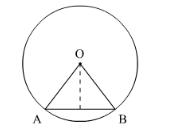# The perimeter of a sector of a circle of radiusQuestion:

The perimeter of a sector of a circle of radius 5.7 m is 27.2 m. Find the area of the sector.

Solution:

We know that the area A of a sector of circle of radius r and arc length l is given by

$A=\frac{1}{2} l r$

Let OAB is the given sector. Then,

Perimeter of sector $O A B=27.2$

$O A+O B+\operatorname{arc} A B=27.2$

$5.7+5.7+\operatorname{arc} A B=27.2$

$11.4+\operatorname{arc} A B=27.21$

$\operatorname{arc} A B=15.8 \mathrm{~m}$

So, $I=15.8 \mathrm{~m}$Now substituting the value of r and l in above formula,

$A=\frac{1}{2} \times 15.8 \times 5.7$

$=45.03 \mathrm{~m}^{2}$